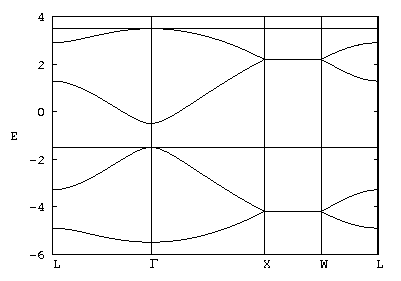Simple Model Band Structure for a Semiconductor

R. B. Laughlin January 19, 2007

(Submitted as coursework for AP272, Stanford University, Winter 2007)Fig. 1: Band structure for the tight binding hamiltonian described in this document with parameters Vh = -1.0 eV and V = -2.5 eV.

This document is a prototype student report for AP272. It is written in plain html and emphasizes ease of reading and good pedagogy over comprehensiveness. Its figure is an embedded 2-color .gif shrunk to a width of 400 pixels for rapid downloading. Clicking on the figure brings up an archival high-resolution version. The computer codes used to generate the figure are included in subdirectories and accessed by downward links. All references are provided - including the origin of the matrix diagonalization routines used. A public domain copyright notice is appended at the end. A prototype tarball of this report is available here

The essence of a band structure for a simple metal or semiconductor is captured elegantly with a tight binding model. The underlying reason is that atomic orbitals are the right degress of freedom for describing electron motion in most solids. We don't use them for high quality calculations, as a rule, because their non-orthogonality is a technical headache to handle. However, tight-binding calculations based on atomic orbitals usually provide the quickest and easiest way to get a rough understanding of the situation.

The most important material to understand by this method is a tetrahedrally bonded semiconductor, such as Si or Ge. The unit cell of such a material contains 2 atoms. The four near neighbors of atom #1 lie at the points of a tetrahedron. All of them are equivalent to atom #2. We shall call the translation vectors going from atom #1 to its four near neighbors &alpha, &beta, &gamma and &delta. The negatives of these translation vectors go atom #2 to its four neighbors. There are 8 important atomic orbitals in the unit cell - one s and three p states for each atom.

We wish to solve the simplest model that gets the physical idea across. Accordingly we first do a unitary transformation to construct hybrid orbitals that point along the four chemical bonds. On atom #1 we have

 h1 = s + px + py + pz h2 = s + px - py - pz h3 = s - px + py - pz h4 = s - px - py + pz

On atom #2 we have

 h5 = s - px - py - pz h6 = s - px + py + pz h7 = s + px - py + pz h8 = s + px + py - pz

We do this because the energy gap is caused by the bonding interaction V between hybrids pointing at each other from adjacent sites. Were this the only interaction in the problem, the two hybrids would mix and spit into bonding and antibonding states separated by energy 2V. These, in turn, would become degenerate valence and conduction bands, which would be flat and degnerate. However, V isn't the only interaction in the problem. The remaining ones broaden out the bands in complex ways. You can model the band structures of all the materials in this class by adjusting the interactions.

Let us now assume that the only interaction other than V is the energy splitting between the s and p orbitals on a given site. We neglected this splitting when we combined them into hybrids. This assumption is oversimplified for Si and Ge, so the bands that result don't match these materials well. However, the qualitative features are right. In the hybrid basis, the s-p splitting becomes a matrix element Vh to tunnel from any hybrid orbital to any other on the same site. With this choice of interaction, the 8 x 8 matrix that we must diagonalize for every crystal momentum q becomes

 0 Vh Vh Vh V eiq·&alpha 0 0 0 Vh 0 Vh Vh 0 V eiq·&beta 0 0 Vh Vh 0 Vh 0 0 V eiq·&gamma 0 Vh Vh Vh 0 0 0 0 V eiq·&delta V e-iq·&alpha 0 0 0 0 Vh Vh Vh 0 V e-iq·&beta 0 0 Vh 0 Vh Vh 0 0 V e-iq·&gamma 0 Vh Vh 0 Vh 0 0 0 V e-iq·&delta Vh Vh Vh 0

While this particular matrix is sufficiently simple that you can obtain some of its eigenvalues by hand, actually doing so is not useful because the mathematical simplicity is an artifact of the parameter choices. It makes more sense to write a computer program that can diagonalize any matrix, and then save the code for later modification to study other sets of parameters. The bands shown in Fig. 1 were generated by such a code, the fortran source of which is available here. The figure itself was generated by gnuplot, the input file of which is available here.

The band structure in Fig. 1 is a sequence of straight-line trajectories between particular values of q, given by

 Γ= (0 , 0, 0) L= π√3/4 b (1 , 1 , 1) X= π√3/2 b (1 , 0 , 0) W= π√3/4 b (2 , 1 , 0)

where b = |α| = ... = |&delta| is the bond length. It consists of four valence bands (E < -1) and four conductions bands (E > -1). The total number of bands is thus the number of orbitals in the unit cell, as expected. The double degeneracy of the flat bands (and also the fact that they are flat) is an artifact of the model that disappears when other interactions are included.

© 2007 R. B. Laughlin. The author grants permission to copy, distribute and display this work in unaltered form, with attribution to the author, for noncommercial purposes only. All other rights, including commercial rights, are reserved to the author.

References

 W. Harrison, Elementary Electronic Structure (World, Singapore, 2004).